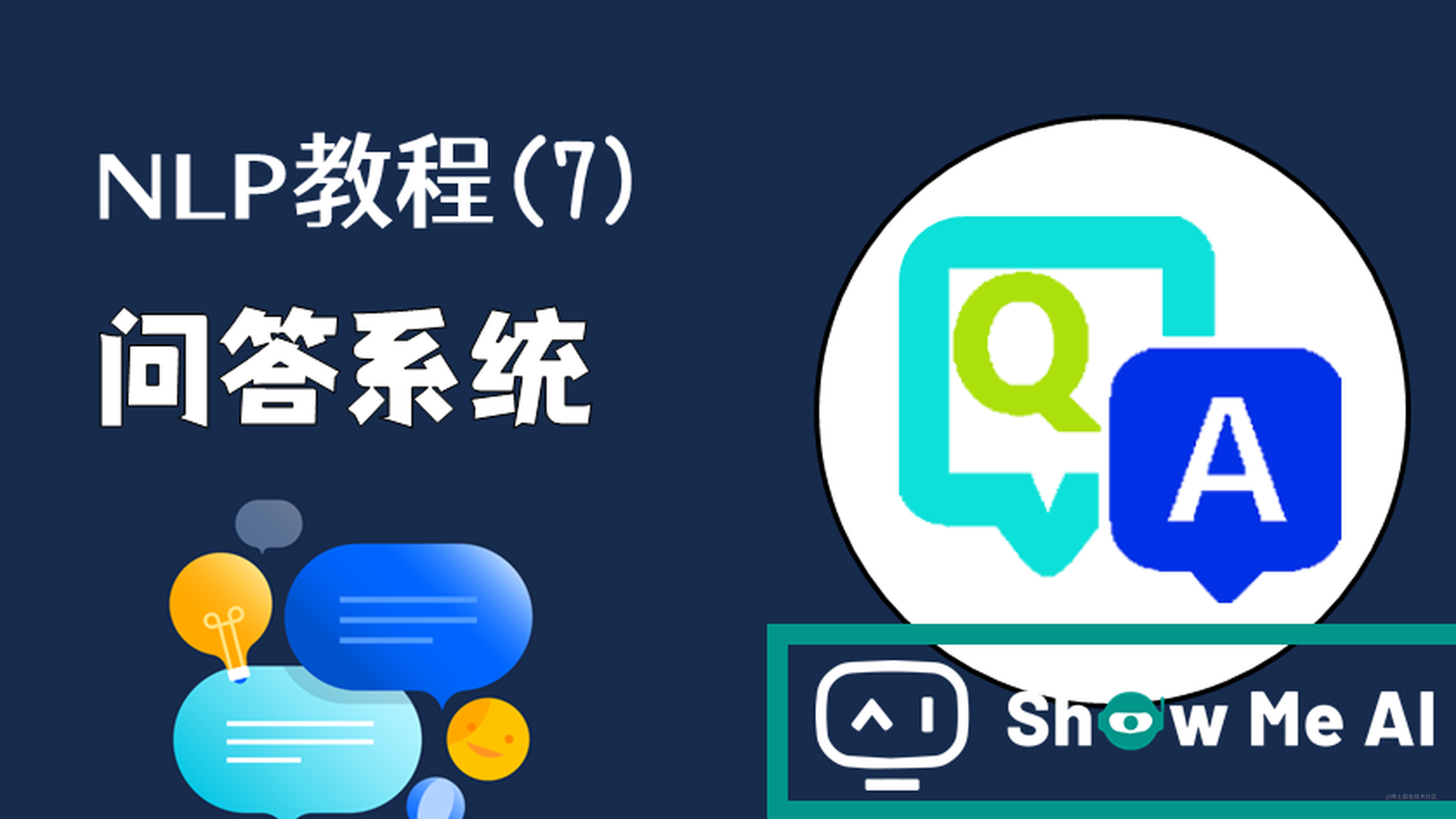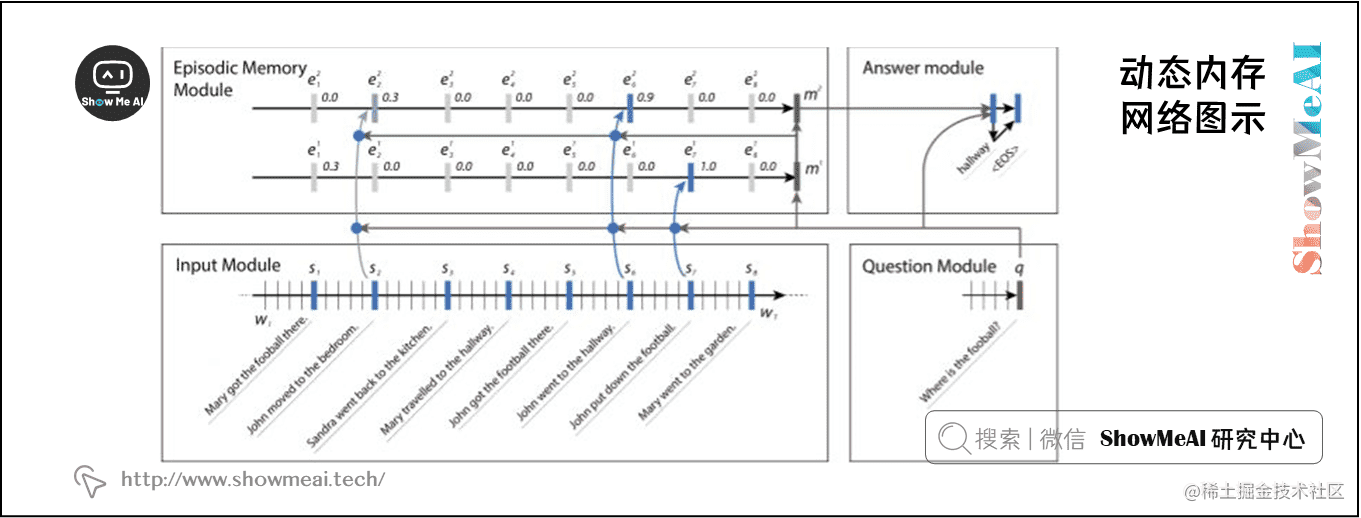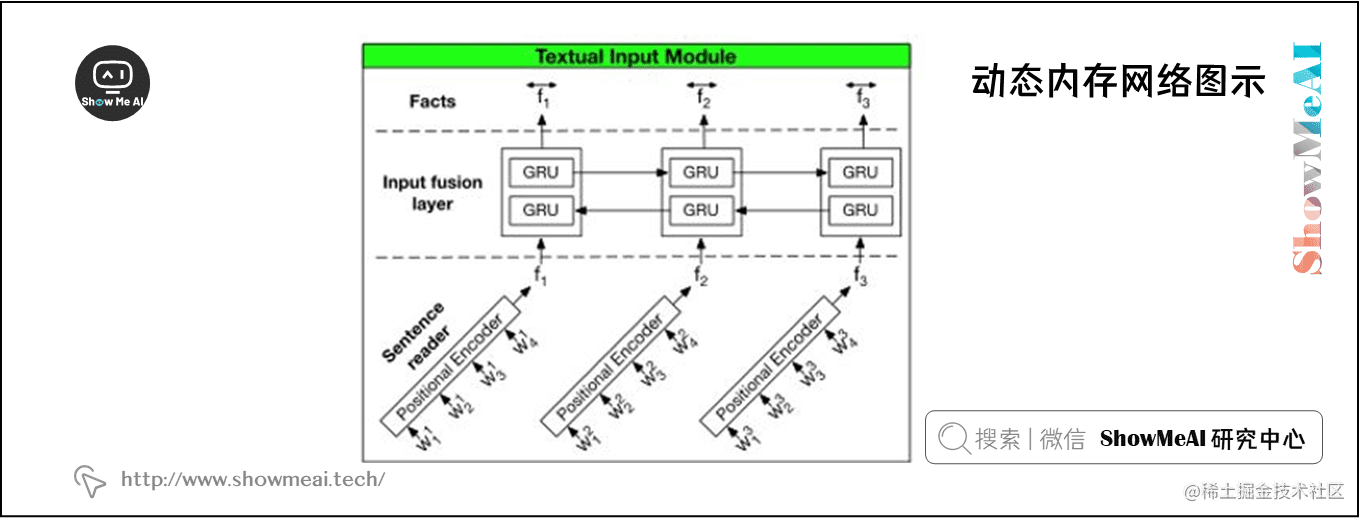# NLP教程(7) - 问答系统本系列为斯坦福CS224n《自然语言处理与深度学习(Natural Language Processing with Deep Learning)》的全套学习笔记，对应的课程视频可以在 这里 查看。ShowMeAI为CS224n课程的全部课件，做了中文翻译和注释，并制作成了 GIF动图！点击 第10讲-NLP中的问答系统 查看的课件注释与带学解读。更多资料获取方式见文末。

# 引言

CS224n是顶级院校斯坦福出品的深度学习与自然语言处理方向专业课程，核心内容覆盖RNN、LSTM、CNN、transformer、bert、问答、摘要、文本生成、语言模型、阅读理解等前沿内容。

## 笔记核心词

• Dynamic Memory Networks \ 动态记忆网络
• QA
• 问答
• 对话
• MemNN
• DCN
• VQA

# 1.图文问答系统与动态记忆网络( DMN )

QA 系统的概念是直接从文档、对话、在线搜索等中提取信息(有时是段落，或是单词的范围)，以满足用户的信息需求。 QA 系统不需要用户通读整个文档，而是倾向于给出一个简短的答案。

• The symbol for mercuric oxide is?(氧化汞的符号是什么？)
• Which NFL team represented the AFC at Super Bowl 50?(哪支NFL球队代表AFC参加超级碗50赛？)

• 我们发出一个查询，然后机器提供一个响应。通过阅读文档或一组指令，智能系统应该能够回答各种各样的问题。
• 我们可以要求句子的 POS 标签，我们可以要求系统用不同的语言来响应。

• ① 许多NLP任务使用不同的架构，如TreeLSTM (Tai et al., 2015)用于情绪分析，Memory Network (Weston et al., 2015) 用于回答问题，以及双向LSTM-CRF (Huang et al., 2015) 用于词性标注。
• ② 全面的多任务学习往往非常困难，迁移学习仍然是当前人工智能领域(计算机视觉、强化学习等)神经网络架构的主要障碍。## 1.1 输入模块（本部分DMN网络频繁使用到GRU结构，具体的GRU细节讲解可以查看ShowMeAI的对吴恩达老师课程的总结文章深度学习教程 | 序列模型与RNN网络，也可以查看本系列的前序文章NLP教程(5) - 语言模型、RNN、GRU与LSTM

## 1.3 情景记忆模块

\begin{aligned} h_{t}^{i} &=g_{t}^{i} \operatorname{GRU}\left(c_{t}, h_{t-1}^{i}\right)+\left(1-g_{t}^{i}\right) h_{t-1}^{i} \\ e_{i} &=h_{T_{\mathrm{C}}}^{i} \end{aligned}

$g_{t}^{i} =G\left(c_{t}, m^{i-1}, q\right)$
$G(c, m, q) =\sigma \left(W^{(2)} tanh \left(W^{(1)} z(c, m, q)+b^{(1)}\right)+b^{(2)}\right)$
$z(c, m, q) =\left[c, m, q, c \circ q, c \circ m,|c-q|,|c-m|, c^{T} W^{(b)} q_{,} c^{T} W^{(b)} m\right]$

## 1.4 回答模块

\begin{aligned} y_{t} &=\operatorname{softmax}\left(W^{(a)} a_{t}\right) \\ a_{t} &=\operatorname{GRU}\left(\left[y_{t-1}, q\right], a_{t-1}\right) \end{aligned}

# 斯坦福 CS224n 课程带学详解